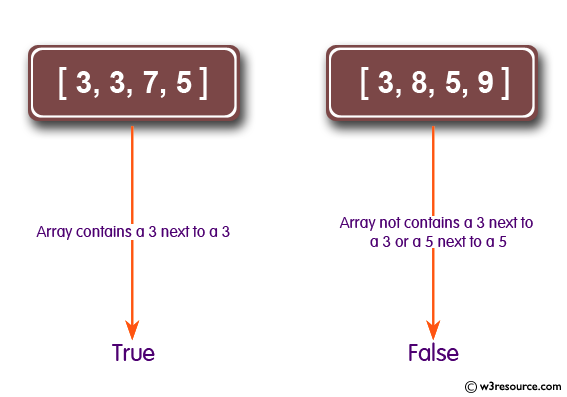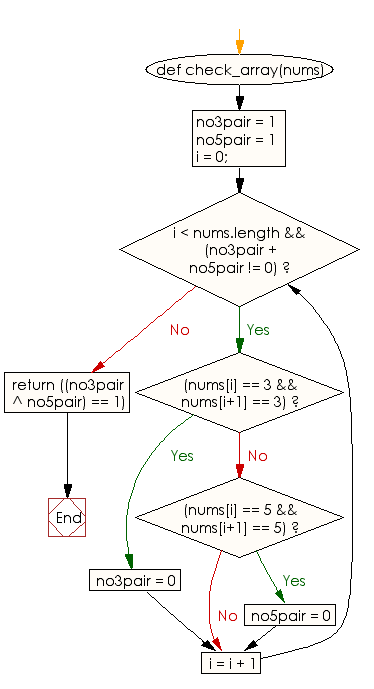﻿ Ruby Array exercises: Check whether a given array contains a 3 next to a 3 or a 5 next to a 5, but not both - w3resource# Ruby Array Exercises: Check whether a given array contains a 3 next to a 3 or a 5 next to a 5, but not both

## Ruby Array: Exercise-38 with Solution

Write a Ruby program to check whether a given array contains a 3 next to a 3 or a 5 next to a 5, but not both.Ruby Code:

``````def check_array(nums)
no3pair = 1
no5pair = 1
i = 0;
while i < nums.length && (no3pair + no5pair != 0)
if(nums[i] == 3 && nums[i+1] == 3)
no3pair = 0
elsif(nums[i] == 5 && nums[i+1] == 5)
no5pair = 0
end
i = i + 1
end
return ((no3pair ^ no5pair) == 1)
end
print check_array([3, 3, 7, 5]),"\n"
print check_array([3, 8, 5, 9]),"\n"
print check_array([3, 7, 5, 5]),"\n"
``````

Output:

```true
false
true
```

Flowchart:Ruby Code Editor: